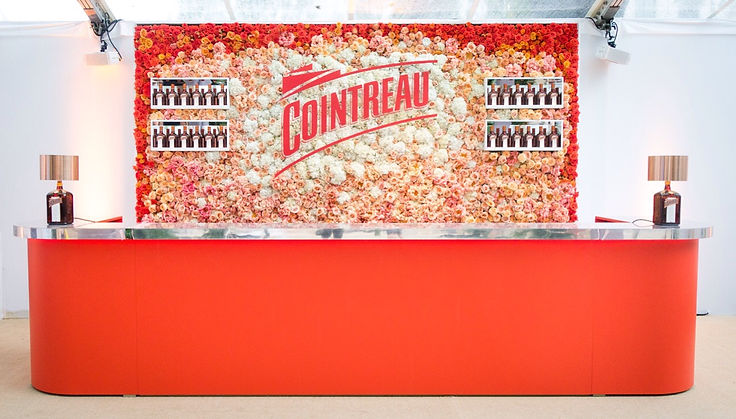J U N_1 8

F A C E B O O K

P H O T O_B O O T H

A G E N C Y: M U S T E R

To play, press and hold the enter key. To stop, release the enter key.

F E B_1 8

T O Y O T A

#G O_Y O U R_O W N_w A Y

A G E N C Y: M U S T E R

T I M E L A P S EJ U N_1 6

C O I N T R E A U_B A R

G L A M O U R_A W A R D S

L O N D O N

A G E N C Y: A C A_L I V ED E C_1 3__J A N_1 4

H E I N E K E N__R O O F T O P

A U C K L A N D